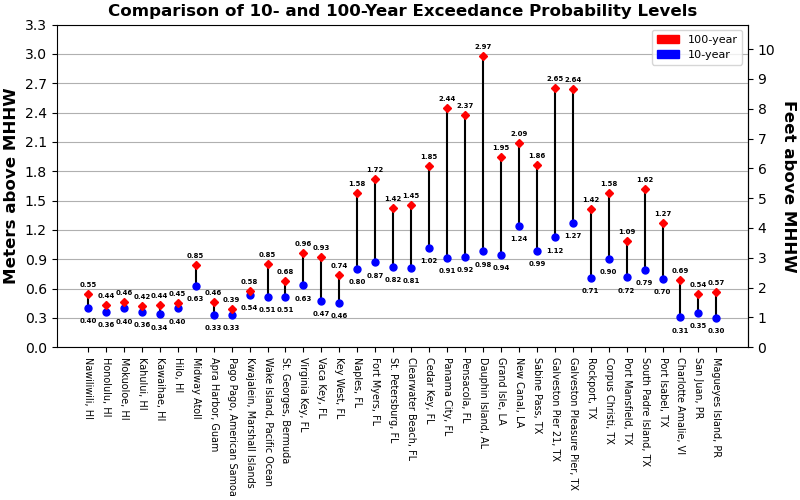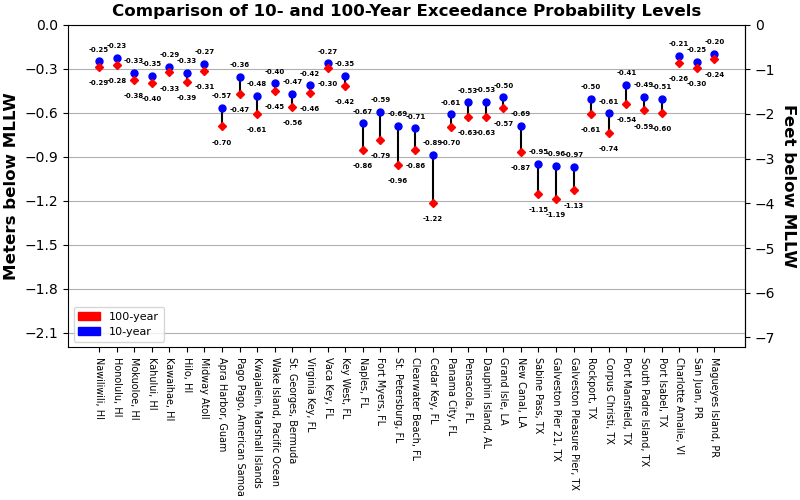### Extreme Exceedance Probability Levels

The graphs compare the 10% and 1% annual exceedance probability levels which correspond to the levels that would be exceeded ten times and one time per century. The high exceedance probability levels are in meters above Mean Higher High Water (MHHW) and the low exceedance probability levels are in meters below Mean Lower Low Water (MLLW).